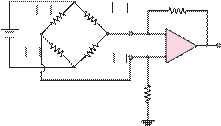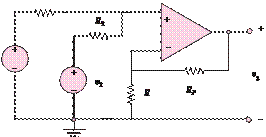### Create an Account

Home / Questions / Show that the circuit of Figure P88 is a noninverting summing amplifier

# Show that the circuit of Figure P88 is a noninverting summing amplifier

Show that the circuit of Figure P8.8 is a noninverting summing amplifier.

10 V

R0 – Ä R

R0 + ÄR100 kÙ

+

R0 + ÄR

R1

R0 – ÄR

R0 = 1 kÙ

-

100 kÙ

voutv1

Jun 18 2020 View more View LessSubscribe To Get Solution